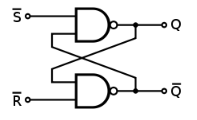Ivan's Homepage# The SR Latches

The SR latch or Set-Reset latch is one of the simplest form of sequential circuits. It is a form of bistable, meaning a circuit with 2 stable states. At first glance, this appear to look like a simple combinational circuit, but don’t let its appearance fool you.Let’s consider what happens when S is 1 and R is 1. Assuming S is 1, Q will be 0 if Q’ is 1; if R is 1, then Q’ will be 1 when Q is 1. Now, let us assume that the circuit starts with Q and Q’ both set to 0. Q will be 1 because Q’ is 0, and Q’ will remain 0 because Q is now 1. We can say at this point that the circuit is “Set”. Then, if we change the input R from 1 to 0 (S remained unchanged), Q’ will become 1, and as a result, Q will become 0.

Here, you notice that the circuit is stable in two different states (Q = 0 or Q = 1). Hence, we’ve created a circuit that “remembers” the state it’s in. In summary, the SR latch has:

• Active low inputs – in other words, the input “activates” when 0 is applied, and remains idle when ‘1’ is applied
• Set = Makes output = 1
• Reset = Makes output = 0

### State analysis

Alright, let’s take a closer look at what’s happening at each stage of the SR latch, i.e. what happens as you change the input, and what actually causes the circuit to latch to a certain state.

An alternative way of creating the latch is by using a pair of NOR gates. The resulting latch has similar characteristics to the NAND version, except for the fact that it has Active High input instead of Active Low inputs. This means that it triggers when the inputs are ‘1’, and remains idle when the input is ‘0’.

Try this yourself with the circuit below: